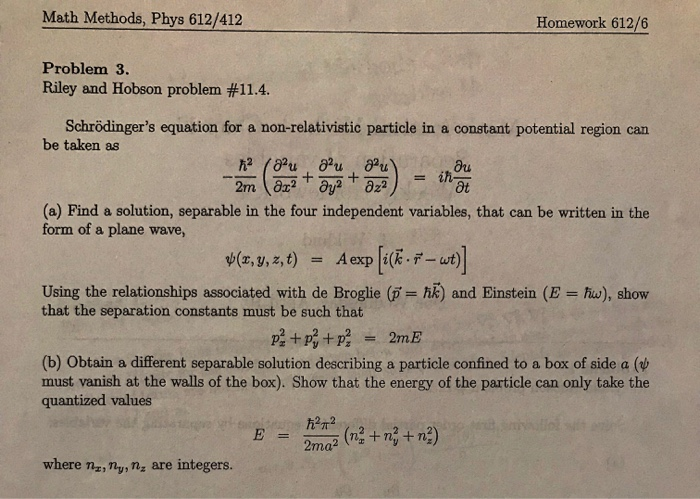# Math Methods, Phys 612/412 Homework 612/6 Problem 3. Riley and Hobson problem 114. Schrödinger's equation for a non-relativistic particle in

###### Question:Math Methods, Phys 612/412 Homework 612/6 Problem 3. Riley and Hobson problem 114. Schrödinger's equation for a non-relativistic particle in a constant potential region can be taken as (a) Find a solution, separable in the four independent variables, that can be written in the form of a plane wave, Aexpli(k-i-cut) ψ(z, y, z, t) Using the relationships associated with de Broglie (ji-M) and Einstein (E that the separation constants must be such that hw), show (b) Obtain a different separable solution describing a particle confined to a box of side a (w must vanish at the walls of the box). Show that the energy of the particle can only take the quantized values where nz,My, ny are integers.